# Pandas太慢？快使用Vaex DataFrame，每秒数亿数据算起来 ?

+关注继续查看? 作者：韩信子@ShowMeAI
? 数据分析实战系列https://www.showmeai.tech/tutorials/40
? 本文地址https://www.showmeai.tech/article-detail/393
? 声明：版权所有，转载请联系平台与作者并注明出处
? 收藏ShowMeAI查看更多精彩内容? Python数据分析实战教程?Vaex 是一个非常强大的 Python DataFrame 库，能够每秒处理数亿甚至数十亿行，而无需将整个数据集加载到内存中。这使得它对于超过单台机器可用 RAM 的大型数据集的探索、可视化和统计分析特别有用，而且 Vaex 还兼具便利性和易用性。# ? vaex 使用详解

## ? 1.巨型文件读取&处理（例如CSV）

Vaex 工具的设计初衷就包括以高效的方式处理比可用内存大得多的文件。借助于它，我们可以轻松处理任意大的数据集。Vaex 在过去的版本中支持二进制文件格式，例如 HDF5、 Arrow 和 Parquet 。从4.14.0版本以来，它也可以像使用上述格式一样轻松打开和使用巨型 CSV 文件。这在一定程度上要归功于 ?Apache Arrow项目，它提供了一个相当高效的 CSV 读取器。print('Check file size on disk:')
!du -h chicago_taxi_2013_2020.csv
print()

df = vaex.open('chicago_taxi_2013_2020.csv')

print(f'Number of rows: {df.shape:,}')
print(f'Number of columns: {df.shape}')

mean_tip_amount = df.tip_amount.mean(progress='widget')
print(f'Mean tip amount: {mean_tip_amount:.2f}')

df.fare_amount.viz.histogram(shape=128, figsize=(6, 4), limits=[0, 42], progress='widget');① 当我们使用vaex.open()对于 CSV 文件，Vaex 将流式处理整个 CSV 文件以确定行数和列数，以及每列的数据类型。这个过程不会占用大量 RAM，但可能需要一些时间，具体取决于 CSV 的行数和列数。

② 然后我们通过 vaex 计算了tip_amount列的平均值，耗时 6 秒。

③ 最后我们绘制了tip_amount列的直方图，耗时 8 秒。

? 注意，无论文件格式如何，Vaex 的 API 都是相同的。这意味着可以轻松地在 CSV、HDF5、Arrow 和 Parquet 文件之间切换，而无需更改代码。

## ? 2.统计：分组聚合

• ① 指定要聚合的列，以及聚合操作的方法名称。
• ② 指定输出列的名称，然后显式实现vaex聚合统计方法。

df = vaex.open('yellow_taxi_2009_2015_f32.hdf5')

print(f'Number of rows: {df.shape:,}')
print(f'Number of columns: {df.shape}')

df.groupby(df.vendor_id, progress='widget').agg(
{'fare_amount': 'mean',                              # Option 1
'tip_amount_mean': vaex.agg.mean(df.tip_amount),    # Option 2
})df.groupby(df.vendor_id, progress='widget').agg(
{'fare_amount_norm': vaex.agg.mean(df.fare_amount) / vaex.agg.std(df.fare_amount)}
)df.groupby(df.vendor_id, progress='widget').agg(
{'fare_amount_mean_all': vaex.agg.mean(df.fare_amount),
'fare_amount_mean_pc2': vaex.agg.mean(df.fare_amount, selection=df.passenger_count == 2),
'fare_amount_mean_pc4': vaex.agg.mean(df.fare_amount, selection=df.passenger_count == 4)}
)## ? 3.进度条

Vaex非常强大，它可以指示每个步骤需要多长时间以及整个管道完成之前还剩下多少时间，在处理巨型文件时，进度条非常有用。

with vaex.progress.tree('rich'):
result_1 = df.groupby(df.passenger_count, agg='count')
result_2 = df.groupby(df.vendor_id, agg=vaex.agg.sum('fare_amount'))
result_3 = df.tip_amount.mean()## ? 4.异步计算

Vaex 具备懒惰计算（lazy computation）的特效，只在必要时计算表达式。一般准则是，对于不改变原始 DataFrame 基本性质的操作，这些操作是惰性计算的。例如：

• 从现有列中创建新列
• 将多个列组合成一个新列
• 进行某种分类编码
• DataFrame 数据过滤

with vaex.progress.tree('rich'):
result_1 = df.groupby(df.passenger_count, agg='count', delay=True)
result_2 = df.groupby(df.vendor_id, agg=vaex.agg.sum('fare_amount'), delay=True)
result_3 = df.tip_amount.mean(delay=True)
df.execute()## ? 5.结果缓存

vaex.cache.on()

with vaex.progress.tree('rich'):
result_1 = df.passenger_count.nunique()

with vaex.progress.tree('rich'):
result_2 = df.groupby(df.passenger_count, agg=vaex.agg.mean('trip_distance'))## ? 6.提前停止

Vaex 有一种直接的方式来确定数据读取的规模，当我们在数据分析时使用 unique, nunique或者 groupby方法，在全量数据上可能会有非常大的时延，我们可以指定 limit参数，它会限制我们计算的范围，从而完成提速。

result = df.pickup_longitude.nunique(limit=100, limit_raise=False)
print(f'result: {result}')## ? 7.云支持

df_cloud = vaex.open('gs://vaex-data/airlines/us_airline_data_1988_2019.hdf5')

print('Size of data:')
print(f'Number of rows: {df_cloud.shape:,}')
print(f'Number of columns: {df_cloud.shape}')

df_cloud.head()## ? 8.GPU加速

print(f'Number of rows: {df.shape:,}')

def arc_distance(theta_1, phi_1, theta_2, phi_2):
temp = (np.sin((theta_2-theta_1)/2*np.pi/180)**2
+ np.cos(theta_1*np.pi/180)*np.cos(theta_2*np.pi/180)
* np.sin((phi_2-phi_1)/2*np.pi/180)**2)
distance = 2 * np.arctan2(np.sqrt(temp), np.sqrt(1-temp))
return distance * 3958.8

df['arc_distance_miles_numpy'] = arc_distance(df.pickup_longitude, df.pickup_latitude,
df.dropoff_longitude, df.dropoff_latitude)

# Requires cupy and NVDIA GPU
df['arc_distance_miles_cuda'] = df['arc_distance_miles_numpy'].jit_cuda()

# Requires metal2 support on MacOS (Apple Silicon and Radeon GPU supported)
# df['arc_distance_miles_metal'] = df['arc_distance_miles_numpy'].jit_metal()

result_cpu = df.arc_distance_miles_numpy.mean(progress='widget')
result_gpu = df.arc_distance_miles_cuda.mean(progress='widget')
print(f'CPU: {result_cpu:.3f} miles')
print(f'GPU: {result_gpu:.3f} miles')# 推荐阅读Python库函数pandas读取excel数据
0 0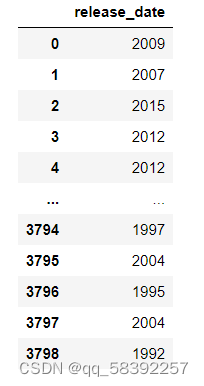pandas使用cut进行分割每组数据
pandas使用cut进行分割每组数据
0 0Pandas 数据重复处理 duplicated()和drop_duplicates()
Pandas 数据重复处理 duplicated()和drop_duplicates()
0 0pandas分批读取CSV并分批处理数据
pandas分批读取CSV并分批处理数据
0 0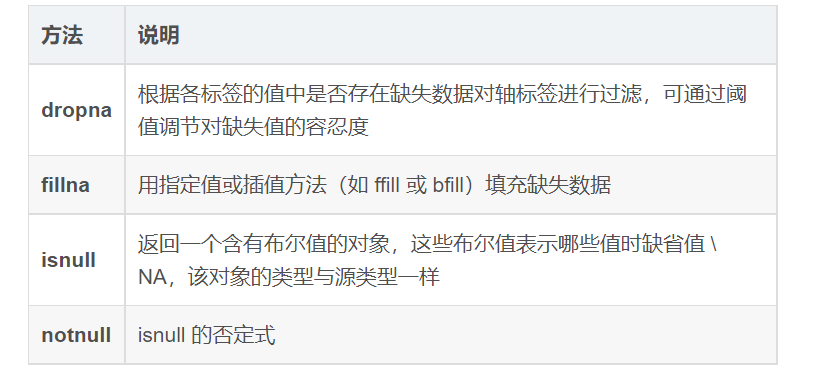Pandas 缺失数据
Pandas 缺失数据
0 0pandas把数据存入mysql
pandas把数据存入mysql
0 0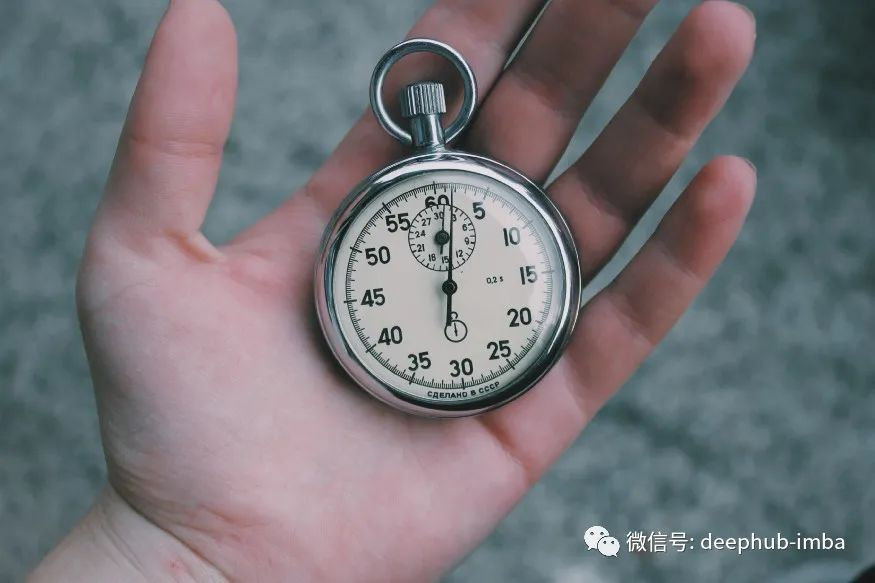0 00 0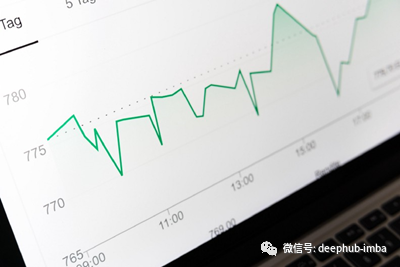Pandas处理时间序列数据的20个关键知识点
Pandas处理时间序列数据的20个关键知识点
0 0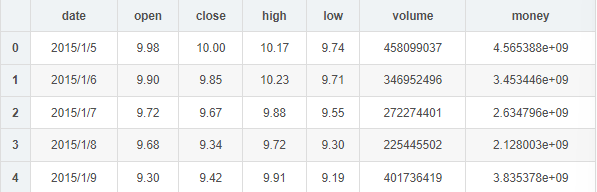0 0
ShowMeAI
『为AI硬核资料库(cool)而生！』构建AI解决方案，用知识加速每一次技术成长！网站：https://www.showmeai.tech/ 公众号：ShowMeAI研究中心